JMSLTM Numerical Library 7.2.0
com.imsl.finance

## Class Bond

• ```public class Bond
extends Object```
Collection of bond functions.

### Definitions

rate is an annualized rate of return based on the par value of the bills.

yield is an annualized rate based on the purchase price and reflects the actual yield to maturity.

coupons are interest payments on a bond.

redemption is the amount a bond pays at maturity.

frequency is the number of times a year that a bond makes interest payments.

basis is the method used to calculate dates. For example, sometimes computations are done assuming 360 days in a year.

issue is the day a bond is first sold.

settlement is the day a purchaser acquires a bond.

maturity is the day a bond's principal is repaid.

### Discount Bonds

Discount bonds, also called zero-coupon bonds, do not pay interest during the life of the security, instead they sell at a discount to their value at maturity. The discount bond methods all have settlement, maturity, basis, and redemption as arguments. In the following list these common arguments are omitted. A related method is `accrintm`, which returns the interest that has accumulated on the discount bond.

### Treasury Bills

US Treasury bills are a special case of discount bonds. The basis is fixed for treasury bills and the redemption value is assumed to be \$100. So these functions have only settlement and maturity as common arguments.

### Interest-Paying Bonds

Most bonds pay interest periodically. The interest-paying bond methods all have settlement, maturity, basis, and frequency as arguments. Again suppressing the common arguments, A related method is `accrint`, which returns the interest that has accumulated at settlement from the previous coupon date.

### Interest-Paying Bonds with Odd Coupon Periods

An odd coupon period is one that is either shorter or longer than the regular coupon period. Odd coupon periods typically occur in the first or the last payment period. The yield and price functions accept two additional arguments in order to make the proper adjustments for odd coupon periods: `issueDate` and `firstCouponDate` or `lastCouponDate`.

### Coupon days

In this diagram, the settlement date is shown as a hollow circle and the adjacent coupon dates are shown as filled circles.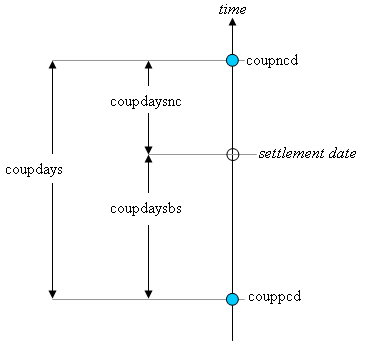A related method is `coupnum`, which returns the number of coupons payable between settlement and maturity.

Another related method is `yearfrac`, which returns the fraction of the year between two days.

### Duration

Duration is used to measure the sensitivity of a bond to changes in interest rates. Convexity is a measure of the sensitivity of duration.
• ### Field Summary

Fields
Modifier and Type Field and Description
`static int` `ANNUAL`
`static int` `BIMONTHLY`
Coupon payments are made bimonthly (6 times per year).
`static int` `MONTHLY`
`static int` `QUARTERLY`
`static int` `SEMIANNUAL`
Coupon payments are made semiannually (twice per year).
• ### Method Summary

Methods
Modifier and Type Method and Description
`static double` ```accrint(GregorianCalendar issue, GregorianCalendar firstCoupon, GregorianCalendar settlement, double rate, double par, int frequency, DayCountBasis basis)```
Returns the interest which has accrued on a security that pays interest periodically.
`static double` ```accrintm(GregorianCalendar issue, GregorianCalendar maturity, double rate, double par, DayCountBasis basis)```
Returns the interest which has accrued on a security that pays interest at maturity.
`static double` ```amordegrc(double cost, GregorianCalendar issue, GregorianCalendar firstPeriod, double salvage, int period, double rate, DayCountBasis basis)```
Returns the depreciation for each accounting period.
`static double` ```amorlinc(double cost, GregorianCalendar issue, GregorianCalendar firstPeriod, double salvage, int period, double rate, DayCountBasis basis)```
Returns the depreciation for each accounting period.
`static double` ```convexity(GregorianCalendar settlement, GregorianCalendar maturity, double coupon, double yield, int frequency, DayCountBasis basis)```
Returns the convexity for a security.
`static int` ```coupdaybs(GregorianCalendar settlement, GregorianCalendar maturity, int frequency, DayCountBasis basis)```
Returns the number of days starting with the beginning of the coupon period and ending with the settlement date.
`static double` ```coupdays(GregorianCalendar settlement, GregorianCalendar maturity, int frequency, DayCountBasis basis)```
Returns the number of days in the coupon period containing the settlement date.
`static int` ```coupdaysnc(GregorianCalendar settlement, GregorianCalendar maturity, int frequency, DayCountBasis basis)```
Returns the number of days starting with the settlement date and ending with the next coupon date.
`static GregorianCalendar` ```coupncd(GregorianCalendar settlement, GregorianCalendar maturity, int frequency, DayCountBasis basis)```
Returns the first coupon date which follows the settlement date.
`static int` ```coupnum(GregorianCalendar settlement, GregorianCalendar maturity, int frequency, DayCountBasis basis)```
Returns the number of coupons payable between the settlement date and the maturity date.
`static GregorianCalendar` ```couppcd(GregorianCalendar settlement, GregorianCalendar maturity, int frequency, DayCountBasis basis)```
Returns the coupon date which immediately precedes the settlement date.
`static double` ```disc(GregorianCalendar settlement, GregorianCalendar maturity, double price, double redemption, DayCountBasis basis)```
Returns the implied interest rate of a discount bond.
`static double` ```duration(GregorianCalendar settlement, GregorianCalendar maturity, double coupon, double yield, int frequency, DayCountBasis basis)```
Returns the Macaulay duration of a security where the security has periodic interest payments.
`static double` ```intrate(GregorianCalendar settlement, GregorianCalendar maturity, double investment, double redemption, DayCountBasis basis)```
Returns the interest rate of a fully invested security.
`static double` ```mduration(GregorianCalendar settlement, GregorianCalendar maturity, double coupon, double yield, int frequency, DayCountBasis basis)```
Returns the modified Macaulay duration for a security with an assumed par value of \$100.
`static double` ```price(GregorianCalendar settlement, GregorianCalendar maturity, double rate, double yield, double redemption, int frequency, DayCountBasis basis)```
Returns the price, per \$100 face value, of a security that pays periodic interest.
`static double` ```price(GregorianCalendar settlement, GregorianCalendar maturity, GregorianCalendar lastCoupon, double rate, double yield, double redemption, int frequency, DayCountBasis basis)```
Returns the price of an odd last period coupon bond, given its yield.
`static double` ```price(GregorianCalendar settlement, GregorianCalendar maturity, GregorianCalendar issueDate, GregorianCalendar firstCoupon, double rate, double yield, double redemption, int frequency, DayCountBasis basis)```
Returns the price of an odd first period, coupon bond, given its yield.
`static double` ```pricedisc(GregorianCalendar settlement, GregorianCalendar maturity, double rate, double redemption, DayCountBasis basis)```
Returns the price of a discount bond given the discount rate.
`static double` ```pricemat(GregorianCalendar settlement, GregorianCalendar maturity, GregorianCalendar issue, double rate, double yield, DayCountBasis basis)```
Returns the price, per \$100 face value, of a discount bond.
`static double` ```priceyield(GregorianCalendar settlement, GregorianCalendar maturity, double yield, double redemption, DayCountBasis basis)```
Returns the price of a discount bond given the yield.
`static double` ```received(GregorianCalendar settlement, GregorianCalendar maturity, double investment, double rate, DayCountBasis basis)```
Returns the amount one receives when a fully invested security reaches the maturity date.
`static double` ```tbilleq(GregorianCalendar settlement, GregorianCalendar maturity, double rate)```
Returns the bond-equivalent yield of a Treasury bill.
`static double` ```tbillprice(GregorianCalendar settlement, GregorianCalendar maturity, double rate)```
Returns the price, per \$100 face value, of a Treasury bill.
`static double` ```tbillyield(GregorianCalendar settlement, GregorianCalendar maturity, double price)```
Returns the yield of a Treasury bill.
`static double` ```yearfrac(GregorianCalendar start, GregorianCalendar end, DayCountBasis basis)```
Returns the fraction of a year represented by the number of whole days between two dates.
`static double` ```yield(GregorianCalendar settlement, GregorianCalendar maturity, double rate, double price, double redemption, int frequency, DayCountBasis basis)```
Returns the yield of a security that pays periodic interest.
`static double` ```yield(GregorianCalendar settlement, GregorianCalendar maturity, GregorianCalendar lastCoupon, double rate, double price, double redemption, int frequency, DayCountBasis basis)```
Returns the yield of a security with an odd last coupon period that pays periodic interest.
`static double` ```yield(GregorianCalendar settlement, GregorianCalendar maturity, GregorianCalendar issueDate, GregorianCalendar firstCoupon, double rate, double price, double redemption, int frequency, DayCountBasis basis)```
Returns the yield of a security with an odd first coupon period that pays periodic interest.
`static double` ```yielddisc(GregorianCalendar settlement, GregorianCalendar maturity, double price, double redemption, DayCountBasis basis)```
Returns the annual yield of a discount bond.
`static double` ```yieldmat(GregorianCalendar settlement, GregorianCalendar maturity, GregorianCalendar issue, double rate, double price, DayCountBasis basis)```
Returns the annual yield of a security that pays interest at maturity.
• ### Methods inherited from class java.lang.Object

`clone, equals, finalize, getClass, hashCode, notify, notifyAll, toString, wait, wait, wait`
• ### Field Detail

• #### ANNUAL

`public static final int ANNUAL`
Constant Field Values
• #### BIMONTHLY

`public static final int BIMONTHLY`
Coupon payments are made bimonthly (6 times per year).
Constant Field Values
• #### MONTHLY

`public static final int MONTHLY`
Constant Field Values
• #### QUARTERLY

`public static final int QUARTERLY`
Constant Field Values
• #### SEMIANNUAL

`public static final int SEMIANNUAL`
Coupon payments are made semiannually (twice per year).
Constant Field Values
• ### Method Detail

• #### accrint

```public static double accrint(GregorianCalendar issue,
GregorianCalendar firstCoupon,
GregorianCalendar settlement,
double rate,
double par,
int frequency,
DayCountBasis basis)```
Returns the interest which has accrued on a security that pays interest periodically. In the equation below, Ai represents the number of days which have accrued for the ith quasi-coupon period within the odd period. The quasi-coupon periods are periods obtained by extending the series of equal payment periods to before or after the actual payment periods. NC represents the number of quasi-coupon periods within the odd period, rounded to the next highest integer. NLi represents the length of the normal ith quasi-coupon period within the odd period. NLi is expressed in days. Function `accrint` can be found by solving the following: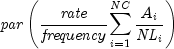Parameters:
`issue` - a `GregorianCalendar` issue date of the security
`firstCoupon` - a `GregorianCalendar` date of the security's first interest date
`settlement` - a `GregorianCalendar` settlement date of the security
`rate` - a `double` which specifies the security's annual coupon rate
`par` - a `double` which specifies the security's par value
`frequency` - an `int` which specifies the number of coupon payments per year: `ANNUAL` for annual, `SEMIANNUAL` for semiannual, and `QUARTERLY` for quarterly
`basis` - a `DayCountBasis` object which contains the type of day count basis to use. See `DayCountBasis`.
Returns:
a `double` which specifies the accrued interest
Example
• #### accrintm

```public static double accrintm(GregorianCalendar issue,
GregorianCalendar maturity,
double rate,
double par,
DayCountBasis basis)```
Returns the interest which has accrued on a security that pays interest at maturity.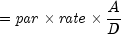In the above equation,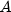represents the number of days starting at issue date to maturity date and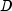represents the annual basis.
Parameters:
`issue` - a `GregorianCalendar` issue date of the security
`maturity` - a `GregorianCalendar` date of the security's maturity
`rate` - a `double` which specifies the security's annual coupon rate
`par` - a `double` which specifies the security's par value
`basis` - a `DayCountBasis` object which contains the type of day count basis to use. See `DayCountBasis`.
Returns:
a `double` which specifies the accrued interest
Example
• #### amordegrc

```public static double amordegrc(double cost,
GregorianCalendar issue,
GregorianCalendar firstPeriod,
double salvage,
int period,
double rate,
DayCountBasis basis)```
Returns the depreciation for each accounting period. This method is similar to `amorlinc`. However, in this method a depreciation coefficient based on the asset life is applied during the evaluation of the function.
Parameters:
`cost` - a `double` which specifies the cost of the asset
`issue` - a `GregorianCalendar` issue date of the asset
`firstPeriod` - a `GregorianCalendar` date of the end of the first period
`salvage` - a `double` which specifies the asset's salvage value at the end of the life of the asset
`period` - an `int` which specifies the period
`rate` - a `double` which specifies the rate of depreciation
`basis` - a `DayCountBasis` object which contains the type of day count basis to use. See `DayCountBasis`.
Returns:
a `double` which specifies the depreciation
Example
• #### amorlinc

```public static double amorlinc(double cost,
GregorianCalendar issue,
GregorianCalendar firstPeriod,
double salvage,
int period,
double rate,
DayCountBasis basis)```
Returns the depreciation for each accounting period. This method is similar to `amordegrc`, except that `amordegrc` has a depreciation coefficient that is applied during the evaluation that is based on the asset life.
Parameters:
`cost` - a `double` which specifes the cost of the asset
`issue` - a `GregorianCalendar` issue date of the asset
`firstPeriod` - a `GregorianCalendar` date of the end of the first period
`salvage` - a `double` which specifies the asset's salvage value at the end of the life of the asset
`period` - an `int` which specifies the period
`rate` - a `double` which specifies the rate of depreciation
`basis` - a `DayCountBasis` object which contains the type of day count basis to use. See `DayCountBasis`.
Returns:
a `double` which specifies the depreciation
Example
• #### convexity

```public static double convexity(GregorianCalendar settlement,
GregorianCalendar maturity,
double coupon,
double yield,
int frequency,
DayCountBasis basis)```
Returns the convexity for a security. Convexity is the sensitivity of the duration of a security to changes in yield. It is computed using the following: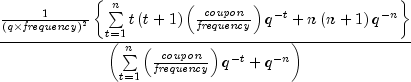where n is calculated from `coupnum`, and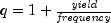.
Parameters:
`settlement` - a `GregorianCalendar` settlement date of the security
`maturity` - a `GregorianCalendar` maturity date of the security
`coupon` - a `double` which specifies the security's annual coupon rate
`yield` - a `double` which specifies the security's annual yield
`frequency` - an `int` which specifies the number of coupon payments per year: `ANNUAL` for annual, `SEMIANNUAL` for semiannual, and `QUARTERLY` for quarterly
`basis` - a `DayCountBasis` object which contains the type of day count basis to use. See `DayCountBasis`.
Returns:
a `double` which specifies the convexity for a security
Example
• #### coupdaybs

```public static int coupdaybs(GregorianCalendar settlement,
GregorianCalendar maturity,
int frequency,
DayCountBasis basis)```
Returns the number of days starting with the beginning of the coupon period and ending with the settlement date. For a good discussion on day count basis, see SIA Standard Securities Calculation Methods 1993, vol. 1, pages 17-35.
Parameters:
`settlement` - a `GregorianCalendar` settlement date of the security
`maturity` - a `GregorianCalendar` maturity date of the security
`frequency` - an `int` which specifies the number of coupon payments per year: `ANNUAL` for annual, `SEMIANNUAL` for semiannual, and `QUARTERLY` for quarterly
`basis` - a `DayCountBasis` object which contains the type of day count basis to use. See `DayCountBasis`.
Returns:
an `int` which specifies the number of days from the beginning of the coupon period to the settlement date
Example
• #### coupdays

```public static double coupdays(GregorianCalendar settlement,
GregorianCalendar maturity,
int frequency,
DayCountBasis basis)```
Returns the number of days in the coupon period containing the settlement date. For a good discussion on day count basis, see SIA Standard Securities Calculation Methods 1993, vol. 1, pages 17-35.
Parameters:
`settlement` - a `GregorianCalendar` settlement date of the security
`maturity` - a `GregorianCalendar` maturity date of the security
`frequency` - an `int` which specifies the number of coupon payments per year: `ANNUAL` for annual, `SEMIANNUAL` for semiannual, and `QUARTERLY` for quarterly
`basis` - a `DayCountBasis` object which contains the type of day count basis to use. See `DayCountBasis`.
Returns:
a `double` which specifies the number of days in the coupon period that contains the settlement date
Example
• #### coupdaysnc

```public static int coupdaysnc(GregorianCalendar settlement,
GregorianCalendar maturity,
int frequency,
DayCountBasis basis)```
Returns the number of days starting with the settlement date and ending with the next coupon date. For a good discussion on day count basis, see SIA Standard Securities Calculation Methods 1993, vol. 1, pages 17-35.
Parameters:
`settlement` - a `GregorianCalendar` settlement date of the security
`maturity` - a `GregorianCalendar` maturity date of the security
`frequency` - an `int` which specifies the number of coupon payments per year: `ANNUAL` for annual, `SEMIANNUAL` for semiannual, and `QUARTERLY` for quarterly
`basis` - a `DayCountBasis` object which contains the type of day count basis to use. See `DayCountBasis`.
Returns:
an `int` which specifies the number of days from the settlement date to the next coupon date
Example
• #### coupncd

```public static GregorianCalendar coupncd(GregorianCalendar settlement,
GregorianCalendar maturity,
int frequency,
DayCountBasis basis)```
Returns the first coupon date which follows the settlement date. For a good discussion on day count basis, see SIA Standard Securities Calculation Methods 1993, vol. 1, pages 17-35.
Parameters:
`settlement` - a `GregorianCalendar` settlement date of the security
`maturity` - a `GregorianCalendar` maturity date of the security
`frequency` - an `int` which specifies the number of coupon payments per year: `ANNUAL` for annual, `SEMIANNUAL` for semiannual, and `QUARTERLY` for quarterly
`basis` - a `DayCountBasis` object which contains the type of day count basis to use. See `DayCountBasis`
Returns:
a `GregorianCalendar` which specifies the next coupon date after the settlement date
Example
• #### coupnum

```public static int coupnum(GregorianCalendar settlement,
GregorianCalendar maturity,
int frequency,
DayCountBasis basis)```
Returns the number of coupons payable between the settlement date and the maturity date. For a good discussion on day count basis, see SIA Standard Securities Calculation Methods 1993, vol. 1, pages 17-35.
Parameters:
`settlement` - a `GregorianCalendar` settlement date of the security
`maturity` - a `GregorianCalendar` maturity date of the security
`frequency` - an `int` which specifies the number of coupon payments per year: `ANNUAL` for annual, `SEMIANNUAL` for semiannual, and `QUARTERLY` for quarterly
`basis` - a `DayCountBasis` object which contains the type of day count basis to use. See `DayCountBasis`.
Returns:
an `int` which specifies the number of coupons payable between the settlement date and maturity date
Example
• #### couppcd

```public static GregorianCalendar couppcd(GregorianCalendar settlement,
GregorianCalendar maturity,
int frequency,
DayCountBasis basis)```
Returns the coupon date which immediately precedes the settlement date. For a good discussion on day count basis, see SIA Standard Securities Calculation Methods 1993, vol. 1, pages 17-35.
Parameters:
`settlement` - a `GregorianCalendar` settlement date of the security
`maturity` - a `GregorianCalendar` maturity date of the security
`frequency` - an `int` which specifies the number of coupon payments per year: `ANNUAL` for annual, `SEMIANNUAL` for semiannual, and `QUARTERLY` for quarterly
`basis` - a `DayCountBasis` object which contains the type of day count basis to use. See `DayCountBasis`
Returns:
a `GregorianCalendar` which specifies the previous coupon date before the settlement date
Example
• #### disc

```public static double disc(GregorianCalendar settlement,
GregorianCalendar maturity,
double price,
double redemption,
DayCountBasis basis)```
Returns the implied interest rate of a discount bond. The discount rate is the interest rate implied when a security is sold for less than its value at maturity in lieu of interest payments. It is computed using the following: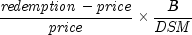In the equation above,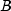represents the number of days in a year based on the annual basis and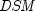represents the number of days starting with the settlement date and ending with the maturity date.
Parameters:
`settlement` - a `GregorianCalendar` settlement date of the security
`maturity` - a `GregorianCalendar` maturity date of the security
`price` - a `double` which specifies the security's price per \$100 face value
`redemption` - a `double` which specifies the security's redemption value per \$100 face value
`basis` - a `DayCountBasis` object which contains the type of day count basis to use. See `DayCountBasis`.
Returns:
a `double` which specifies the discount rate for a security
Example
• #### duration

```public static double duration(GregorianCalendar settlement,
GregorianCalendar maturity,
double coupon,
double yield,
int frequency,
DayCountBasis basis)```
Returns the Macaulay duration of a security where the security has periodic interest payments. The Macaulay duration is the weighted-average time to the payments, where the weights are the present value of the payments. It is computed using the following: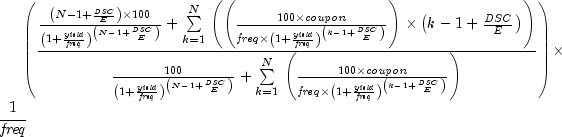In the equation above,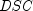represents the number of days starting with the settlement date and ending with the next coupon date.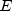represents the number of days within the coupon period.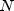represents the number of coupons payable from the settlement date to the maturity date.represents the frequency of the coupon payments annually.
Parameters:
`settlement` - a `GregorianCalendar` settlement date of the security
`maturity` - a `GregorianCalendar` maturity date of the security
`coupon` - a `double` which specifies the security's annual coupon rate
`yield` - a `double` which specifies the security's annual yield
`frequency` - an `int` which specifies the number of coupon payments per year: `ANNUAL` for annual, `SEMIANNUAL` for semiannual, and `QUARTERLY` for quarterly
`basis` - a `DayCountBasis` object which contains the type of day count basis to use. See `DayCountBasis`.
Returns:
a `double` which specifies the annual duration of a security with periodic interest payments
Example
• #### intrate

```public static double intrate(GregorianCalendar settlement,
GregorianCalendar maturity,
double investment,
double redemption,
DayCountBasis basis)```
Returns the interest rate of a fully invested security. It is computed using the following: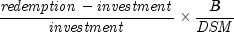In the equation above,represents the number of days in a year based on the annual basis, andrepresents the number of days in the period starting with the settlement date and ending with the maturity date.
Parameters:
`settlement` - a `GregorianCalendar` settlement date of the security
`maturity` - a `GregorianCalendar` maturity date of the security
`investment` - a `double` which specifies the amount invested
`redemption` - a `double` which specifies the amount to be received at maturity
`basis` - a `DayCountBasis` object which contains the type of day count basis to use. See `DayCountBasis`.
Returns:
a `double` which specifies the interest rate for a fully invested security
Example
• #### mduration

```public static double mduration(GregorianCalendar settlement,
GregorianCalendar maturity,
double coupon,
double yield,
int frequency,
DayCountBasis basis)```
Returns the modified Macaulay duration for a security with an assumed par value of \$100. It is computed using the following: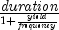where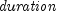is calculated from `mduration`.
Parameters:
`settlement` - a `GregorianCalendar` settlement date of the security
`maturity` - a `GregorianCalendar` maturity date of the security
`coupon` - a `double` which specifies the security's annual coupon rate
`yield` - a `double` which specifies the security's annual yield
`frequency` - an `int` which specifies the number of coupon payments per year: `ANNUAL` for annual, `SEMIANNUAL` for semiannual, and `QUARTERLY` for quarterly
`basis` - a `DayCountBasis` object which contains the type of day count basis to use. See `DayCountBasis`.
Returns:
a `double` which specifies the modified Macaulay duration for a security with an assumed par value of \$100
Example
• #### price

```public static double price(GregorianCalendar settlement,
GregorianCalendar maturity,
double rate,
double yield,
double redemption,
int frequency,
DayCountBasis basis)```
Returns the price, per \$100 face value, of a security that pays periodic interest. It is computed using the following: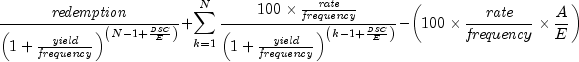In the above equation,represents the number of days in the period starting with the settlement date and ending with the next coupon date.represents the number of days within the coupon period.represents the number of coupons payable in the timeframe from the settlement date to the redemption date.represents the number of days in the timeframe starting with the beginning of coupon period and ending with the settlement date.
Parameters:
`settlement` - a `GregorianCalendar` settlement date of the security
`maturity` - a `GregorianCalendar` maturity date of the security
`rate` - a `double` which specifies the security's annual coupon rate
`yield` - a `double` which specifies the security's annual yield
`redemption` - a `double` which specifies the security's redemption value per \$100 face value
`frequency` - an `int` which specifies the number of coupon payments per year: `ANNUAL` for annual, `SEMIANNUAL` for semiannual, and `QUARTERLY` for quarterly
`basis` - a `DayCountBasis` object which contains the type of day count basis to use. See `DayCountBasis`.
Returns:
a `double` which specifies the price per \$100 face value of a security that pays periodic interest
Example
• #### price

```public static double price(GregorianCalendar settlement,
GregorianCalendar maturity,
GregorianCalendar lastCoupon,
double rate,
double yield,
double redemption,
int frequency,
DayCountBasis basis)```
Returns the price of an odd last period coupon bond, given its yield.

In the case of a short last period with one coupon period or less to redemption, the following formula is used: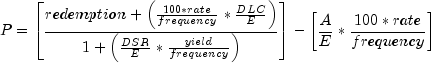where

Variable Description
A Number of days from last coupon date before redemption to settlement date.
DLC Number of days from last coupon date before redemption to redemption.
DSR Number of days from settlement date to redemption date.
E Number of days in the quasi-coupon period.

In the case of a short last period with more than one coupon period to redemption, the following formula is used: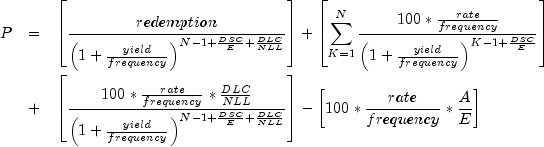where

Variable Description
A Number of days from beginning of coupon period to settlement date.
DLC Number of days from last coupon date before redemption to redemption.
DSC Number of days from settlement date to next coupon date.
E Number of days in coupon period in which the settlement date falls.
N Number of coupons payable between settlement date and last coupon date before redemption.
NLL Number of days in the full quasi-coupon period in which the odd last period falls.

In the case of a long last period with one coupon period or less to redemption, the following formula is used: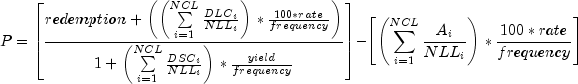where

Variable Description
Ai Number of accrued days for the ith quasi-coupon period within the odd period counting forward from the last interest date before redemption.
DLCi Number of days in the ith quasi-coupon period as delimited by the length of the actual coupon period.
DSCi Number of days from settlement date (or beginning of quasi-coupon period) to next quasi-coupon within odd period (or to redemption date) for the ith quasi-coupon period.
NCL Number of quasi-coupon periods that fit in odd period.
NLLi Normal length in days of the full ith quasi-coupon period within odd last period.

In the case of a long first period with more than one coupon period to redemption, the following formula is used: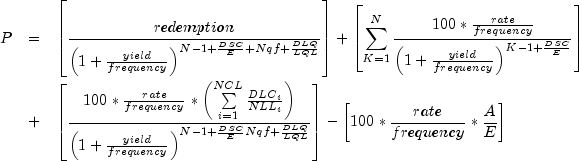where

Variable Description
A Number of days from beginning of coupon period to settlement date.
DLCi Number of days from last coupon date before redemption to first quasi-coupon or number of days in quasi-coupon.
DLQ Number of days counted in the last quasi-coupon period within the last odd period.
DSC Number of days from settlement date to next coupon date.
E Number of days in coupon period in which the settlement falls.
LQL Normal length in days of the last quasi-coupon period within the odd last period.
N Number of coupons payable between settlement date and last coupon date before redemption.
NCL Number of quasi-coupon periods that fit in odd period.
NLLi Normal length in days of the full ith quasi-coupon period within odd last period.
Nql Number of whole quasi-coupon periods between last coupon date before redemption and the redemption date.
Parameters:
`settlement` - a `GregorianCalendar` settlement date of the security
`maturity` - a `GregorianCalendar` maturity date of the security
`lastCoupon` - a `GregorianCalendar` last interest date of the security
`rate` - a `double` which specifies the security's annual coupon rate
`yield` - a `double` which specifies the security's annual yield
`redemption` - a `double` which specifies the security's redemption value per \$100 face value
`frequency` - an `int` which specifies the number of coupon payments per year: `ANNUAL` for annual, `SEMIANNUAL` for semiannual, and `QUARTERLY` for quarterly
`basis` - a `DayCountBasis` object which contains the type of day count basis to use. See `DayCountBasis`.
Returns:
a `double` that indicates the bond price.
Example 1, Example 2, Example 3, Example 4
• #### price

```public static double price(GregorianCalendar settlement,
GregorianCalendar maturity,
GregorianCalendar issueDate,
GregorianCalendar firstCoupon,
double rate,
double yield,
double redemption,
int frequency,
DayCountBasis basis)```
Returns the price of an odd first period, coupon bond, given its yield.

In the case of a short first period, the following formula is used: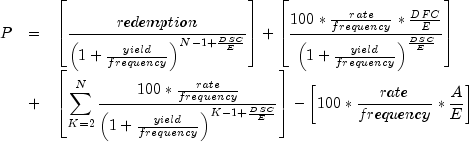where

Variable Description
A Number of days from the beginning of coupon period to the settlement date (accrued days).
DFC Number of days from the beginning of odd first coupon period (`issueDate`) to the first coupon date.
DSC Number of days from the settlement date to first coupon date.
E Number of days in the quasi-coupon period in which the settlement date falls.
N Number of coupons payable between settlement date and redemption date.

In the case of a long first period, the following formula is used: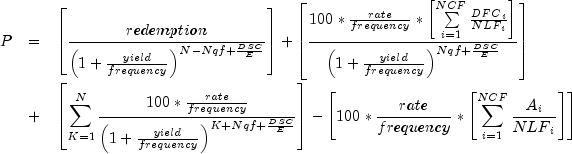where

Variable Description
Ai Number of accrued days for the ith quasi-coupon period within the odd period.
DFCi Number of days from the `issueDate` to the first quasi-coupon or number of days in the the quasi-coupon.
DSC Number of days from the settlement date to the next coupon or quasi-coupon date.
E Number of days in the quasi-coupon or coupon period in which the settlement date falls.
N Number of coupon periods between the first real coupon date and redemption date.
NCF Number of quasi-coupon periods that fall in the odd period.
NLFi Number of days in the full ith quasi-coupon period within the odd period.
Nqf Number of whole quasi-coupon periods between `settlement` and `firstCoupon`.
Parameters:
`settlement` - a `GregorianCalendar` settlement date of the security
`maturity` - a `GregorianCalendar` maturity date of the security
`issueDate` - a `GregorianCalendar` issue date of the security
`firstCoupon` - a `GregorianCalendar` first coupon date of the security
`rate` - a `double` which specifies the security's annual coupon rate
`yield` - a `double` which specifies the security's annual yield
`redemption` - a `double` which specifies the security's redemption value per \$100 face value
`frequency` - an `int` which specifies the number of coupon payments per year: `ANNUAL` for annual, `SEMIANNUAL` for semiannual, and `QUARTERLY` for quarterly
`basis` - a `DayCountBasis` object which contains the type of day count basis to use. See `DayCountBasis`.
Returns:
a `double` that indicates the bond price.
Example 1, Example 2
• #### pricedisc

```public static double pricedisc(GregorianCalendar settlement,
GregorianCalendar maturity,
double rate,
double redemption,
DayCountBasis basis)```
Returns the price of a discount bond given the discount rate. It is computed using the following: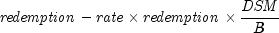In the equation above,represents the number of days starting at the settlement date and ending with the maturity date.represents the number of days in a year based on the annual basis.
Parameters:
`settlement` - a `GregorianCalendar` settlement date of the security
`maturity` - a `GregorianCalendar` maturity date of the security
`rate` - a `double` which specifies the security's discount rate
`redemption` - a `double` which specifies the security's redemption value per \$100 face value
`basis` - a `DayCountBasis` object which contains the type of day count basis to use. See `DayCountBasis`.
Returns:
a `double` which specifies the price per \$100 face value of a discounted security
Example
• #### pricemat

```public static double pricemat(GregorianCalendar settlement,
GregorianCalendar maturity,
GregorianCalendar issue,
double rate,
double yield,
DayCountBasis basis)```
Returns the price, per \$100 face value, of a discount bond. It is computed using the following: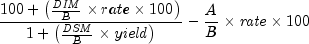In the equation above,represents the number of days in a year based on the annual basis.represents the number of days in the period starting with the settlement date and ending with the maturity date.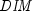represents the number of days in the period starting with the issue date and ending with the maturity date.represents the number of days in the period starting with the issue date and ending with the settlement date.
Parameters:
`settlement` - a `GregorianCalendar` settlement date of the security
`maturity` - a `GregorianCalendar` maturity date of the security
`issue` - a `GregorianCalendar` issue date of the security
`rate` - a `double` which specifies the security's interest rate at issue date
`yield` - a `double` which specifies the security's annual yield
`basis` - a `DayCountBasis` object which contains the type of day count basis to use. See `DayCountBasis`.
Returns:
a `double` which specifies the price per \$100 face value of a security that pays interest at maturity
Example
• #### priceyield

```public static double priceyield(GregorianCalendar settlement,
GregorianCalendar maturity,
double yield,
double redemption,
DayCountBasis basis)```
Returns the price of a discount bond given the yield. It is computed using the following: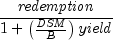In the equation above,represents the number of days starting at the settlement date and ending with the maturity date.represents the number of days in a year based on the annual basis.
Parameters:
`settlement` - a `GregorianCalendar` settlement date of the security
`maturity` - a `GregorianCalendar` maturity date of the security
`yield` - a `double` which specifies the security's yield
`redemption` - a `double` which specifies the security's redemption value per \$100 face value
`basis` - a `DayCountBasis` object which contains the type of day count basis to use. See `DayCountBasis`
Returns:
a `double` which specifies the price per \$100 face value of a discounted security
Example

```public static double received(GregorianCalendar settlement,
GregorianCalendar maturity,
double investment,
double rate,
DayCountBasis basis)```
Returns the amount one receives when a fully invested security reaches the maturity date. It is computed using the following: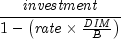In the equation above,represents the number of days in a year based on the annual basis, andrepresents the number of days in the period starting with the issue date and ending with the maturity date.
Parameters:
`settlement` - a `GregorianCalendar` settlement date of the security
`maturity` - a `GregorianCalendar` maturity date of the security
`investment` - a `double` which specifies the amount invested in the security
`rate` - a `double` which specifies the security's rate at issue date
`basis` - a `DayCountBasis` object which contains the type of day count basis to use. See `DayCountBasis`.
Returns:
a `double` which specifies the amount received at maturity for a fully invested security
Example
• #### tbilleq

```public static double tbilleq(GregorianCalendar settlement,
GregorianCalendar maturity,
double rate)```
Returns the bond-equivalent yield of a Treasury bill. It is computed using the following:

If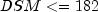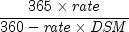otherwise,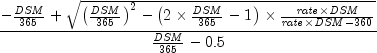In the above equation,represents the number of days starting at settlement date to maturity date.
Parameters:
`settlement` - a `GregorianCalendar` settlement date of the Treasury bill
`maturity` - a `GregorianCalendar` maturity date of the Treasury bill. The maturity cannot be more than a year after the settlement.
`rate` - a `double` which specifies the Treasury bill's discount rate at issue date. The discount rate is an annualized rate of return based on the par value of the bills. The discount rate is calculated on a 360-day basis (twelve 30-day months).
Returns:
a `double` which specifies the bond-equivalent yield for the Treasury bill. This is an annualized rate based on the purchase price of the bills and reflects the actual yield to maturity.
Example
• #### tbillprice

```public static double tbillprice(GregorianCalendar settlement,
GregorianCalendar maturity,
double rate)```
Returns the price, per \$100 face value, of a Treasury bill. It is computed using the following: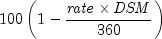In the equation above,represents the number of days in the period starting with the settlement date and ending with the maturity date (any maturity date that is more than one calendar year after the settlement date is excluded).
Parameters:
`settlement` - a `GregorianCalendar` settlement date of the Treasury bill
`maturity` - a `GregorianCalendar` maturity date of the Treasury bill. The maturity cannot be more than a year after the settlement
`rate` - a `double` which specifies the Treasury bill's discount rate at issue date. The discount rate is an annualized rate of return based on the par value of the bills. The discount rate is calculated on a 360-day basis (twelve 30-day months).
Returns:
a `double` which specifies the price per \$100 face value for the Treasury bill
Example
• #### tbillyield

```public static double tbillyield(GregorianCalendar settlement,
GregorianCalendar maturity,
double price)```
Returns the yield of a Treasury bill. It is computed using the following: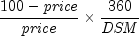In the equation above,represents the number of days in the period starting with the settlement date and ending with the maturity date (any maturity date that is more than one calendar year after the settlement date is excluded).
Parameters:
`settlement` - a `GregorianCalendar` settlement date of the Treasury bill
`maturity` - a `GregorianCalendar` maturity date of the Treasury bill. The maturity cannot be more than a year after the settlement.
`price` - a `double` which specifies the Treasury bill's price per \$100 face value
Returns:
a `double` which specifies the yield for the Treasury bill. This is an annualized rate based on the purchase price of the bills and reflects the actual yield to maturity.
Example
• #### yearfrac

```public static double yearfrac(GregorianCalendar start,
GregorianCalendar end,
DayCountBasis basis)```
Returns the fraction of a year represented by the number of whole days between two dates. It is computed using the following: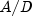whereequals the number of days from `start` to `end`,equals annual basis.
Parameters:
`start` - a `GregorianCalendar` start date of the security
`end` - a `GregorianCalendar` end date of the security
`basis` - a `DayCountBasis` object which contains the type of day count basis to use. See `DayCountBasis`.
Returns:
a `double` which specifies the annual yield of a security that pays interest at maturity
Example
• #### yield

```public static double yield(GregorianCalendar settlement,
GregorianCalendar maturity,
double rate,
double price,
double redemption,
int frequency,
DayCountBasis basis)```
Returns the yield of a security that pays periodic interest. If there is one coupon period, use the following: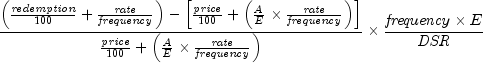In the equation above,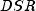represents the number of days in the period starting with the settlement date and ending with the redemption date.represents the number of days within the coupon period.represents the number of days in the period starting with the beginning of coupon period and ending with the settlement date.

If there is more than one coupon period, use the following: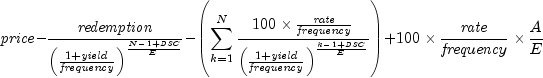In the equation above,represents the number of days in the period from the settlement to the next coupon date.represents the number of days within the coupon period.represents the number of coupons payable in the period starting with the settlement date and ending with the redemption date.represents the number of days in the period starting with the beginning of the coupon period and ending with the settlement date.
Parameters:
`settlement` - a `GregorianCalendar` settlement date of the security
`maturity` - a `GregorianCalendar` maturity date of the security
`rate` - a `double` which specifies the security's annual coupon rate
`price` - a `double` which specifies the security's price per \$100 face value
`redemption` - a `double` which specifies the security's redemption value per \$100 face value
`frequency` - an `int` which specifies the number of coupon payments per year: `ANNUAL` for annual, `SEMIANNUAL` for semiannual, and `QUARTERLY` for quarterly
`basis` - a `DayCountBasis` object which contains the type of day count basis to use. See `DayCountBasis`.
Returns:
a `double` which specifies the yield of a security that pays periodic interest
Example
• #### yield

```public static double yield(GregorianCalendar settlement,
GregorianCalendar maturity,
GregorianCalendar lastCoupon,
double rate,
double price,
double redemption,
int frequency,
DayCountBasis basis)```
Returns the yield of a security with an odd last coupon period that pays periodic interest.

The yield is determined by finding the zero of the function desired price - computed price, where computed price is the output of ```price(java.util.GregorianCalendar, java.util.GregorianCalendar, java.util.GregorianCalendar, double, double, double, int, com.imsl.finance.DayCountBasis)```.

Parameters:
`settlement` - a `GregorianCalendar` settlement date of the security
`maturity` - a `GregorianCalendar` maturity date of the security
`lastCoupon` - a `GregorianCalendar` last coupon date of the security
`rate` - a `double` which specifies the security's annual coupon rate
`price` - a `double` which specifies the security's price per \$100 face value
`redemption` - a `double` which specifies the security's redemption value per \$100 face value
`frequency` - an `int` which specifies the number of coupon payments per year: `ANNUAL` for annual, `SEMIANNUAL` for semiannual and `QUARTERLY` for quarterly
`basis` - a `DayCountBasis` object which contains the type of day count basis to use. See `DayCountBasis`.
Returns:
a `double` which specifies the yield of a security that pays periodic interest
Example 1, Example 2, Example 3, Example 4
• #### yield

```public static double yield(GregorianCalendar settlement,
GregorianCalendar maturity,
GregorianCalendar issueDate,
GregorianCalendar firstCoupon,
double rate,
double price,
double redemption,
int frequency,
DayCountBasis basis)```
Returns the yield of a security with an odd first coupon period that pays periodic interest.

The yield is determined by finding the zero of the function desired price - computed price, where computed price is the output of ```price(java.util.GregorianCalendar, java.util.GregorianCalendar, java.util.GregorianCalendar, java.util.GregorianCalendar, double, double, double, int, com.imsl.finance.DayCountBasis)```.

Parameters:
`settlement` - a `GregorianCalendar` settlement date of the security
`maturity` - a `GregorianCalendar` maturity date of the security
`issueDate` - a `GregorianCalendar` issueDate date of the security
`firstCoupon` - a `GregorianCalendar` first coupon date of the security
`rate` - a `double` which specifies the security's annual coupon rate
`price` - a `double` which specifies the security's price per \$100 face value
`redemption` - a `double` which specifies the security's redemption value per \$100 face value
`frequency` - an `int` which specifies the number of coupon payments per year: `ANNUAL` for annual, `SEMIANNUAL` for semiannual, and `QUARTERLY` for quarterly
`basis` - a `DayCountBasis` object which contains the type of day count basis to use. See `DayCountBasis`.
Returns:
a `double` which specifies the yield of a security that pays periodic interest
Example 1, Example 2
• #### yielddisc

```public static double yielddisc(GregorianCalendar settlement,
GregorianCalendar maturity,
double price,
double redemption,
DayCountBasis basis)```
Returns the annual yield of a discount bond. It is computed using the following:In the equation above,represents the number of days in a year based on the annual basis, andrepresents the number of days starting with the settlement date and ending with the maturity date.
Parameters:
`settlement` - a `GregorianCalendar` settlement date of the security
`maturity` - a `GregorianCalendar` maturity date of the security
`price` - a `double` which specifies the security's price per \$100 face value
`redemption` - a `double` which specifies the security's redemption value per \$100 face value
`basis` - a `DayCountBasis` object which contains the type of day count basis to use. See `DayCountBasis`.
Returns:
a `double` which specifies the annual yield for a discounted security
Example
• #### yieldmat

```public static double yieldmat(GregorianCalendar settlement,
GregorianCalendar maturity,
GregorianCalendar issue,
double rate,
double price,
DayCountBasis basis)```
Returns the annual yield of a security that pays interest at maturity. It is computed using the following: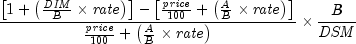In the equation above,represents the number of days in the period starting with the issue date and ending with the maturity date.represents the number of days in the period starting with the settlement date and ending with the maturity date.represents the number of days in the period starting with the issue date and ending with the settlement date.represents the number of days in a year based on the annual basis.
Parameters:
`settlement` - a `GregorianCalendar` settlement date of the security
`maturity` - a `GregorianCalendar` maturity date of the security
`issue` - a `GregorianCalendar` issue date of the security
`rate` - a `double` which specifies the security's interest rate at date of issue
`price` - a `double` which specifies the security's price per \$100 face value
`basis` - a `DayCountBasis` object which contains the type of day count basis to use. See `DayCountBasis`.
Returns:
a `double` which specifies the annual yield of a security that pays interest at maturity